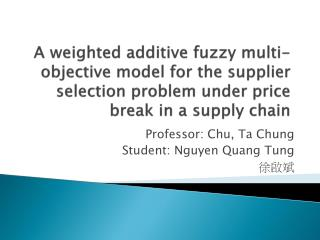DownloadDownload PresentationProfessor: Chu, Ta Chung Student: Nguyen Quang Tung 徐啟斌

# Professor: Chu, Ta Chung Student: Nguyen Quang Tung 徐啟斌

Télécharger la présentation## Professor: Chu, Ta Chung Student: Nguyen Quang Tung 徐啟斌

- - - - - - - - - - - - - - - - - - - - - - - - - - - E N D - - - - - - - - - - - - - - - - - - - - - - - - - - -
##### Presentation Transcript

1. A weighted additive fuzzy multi-objective model for the supplier selection problem under price break in a supply chain Professor: Chu, Ta Chung Student: Nguyen Quang Tung 徐啟斌

2. Introduction • Supplier selection is one of the most critical activities of purchasing management in a supply chain, because of the key role of supplier’s performance on cost, quality, delivery and service in achieving the objectives of a supply chain. • Supplier selection is a multiple-criteria decision-making (MCDM) problem that is affected by several conflicting factors. Depending on the purchasing situations, criteria have varying importance and there is a need to weight criteria. • In practice, for supplier selection problems, most of the input information is not known precisely. In these cases, the theory of fuzzy sets is one of the best tools for handling uncertainty. The fuzzy multi-objective model is formulated in such a way as to simultaneously consider the imprecision of information and determine the order quantities to each supplier based on price breaks.

3. Introduction (cont’) • The problem includes the three objective functions: minimizing the net cost, minimizing the net rejected items and minimizing the net late deliveries, while satisfying capacity and demand requirement constraints. • In order to solve the problem, a fuzzy weighted additive and mixed integer linear programming is developed. • The model aggregates weighted membership functions of objectives to construct the relevant decision functions, in which objectives have different relative importance.

4. An integrated multi-objective supplier selection • Notation definition: • xij: the number of units purchased from the ith supplier at price level j • Pij: price of the ithsupplier at level j • Vij: maximum purchased volume from the ith supplier at jthprice level • D: demand over the period • V*ij: slightly less than Vij • mi: number of price level of the ith supplier • Yij: integer variable for the ithsupplier at jth price level • Ci: capacity of the ith supplier • Fi: percentage of items delivered late for the ith supplier • Si: percentage of rejected units for the ith supplier • n: number of suppliers

5. An integrated multi-objective supplier selection • Objective functions:

6. An integrated multi-objective supplier selection • Constraints At most one price level per supplier can be chosen

7. A fuzzy multi-objective programming • The model is proposed by Zimmermann (1978) with the purpose of finding a vector xT = [x1, x2, …, xn] to satisfy Subject to For fuzzy constraints For deterministic constraints

8. A fuzzy multi-objective programming (cont’) • The linear membership function for minimization goals is obtained through solving the multi-objective problem as a single objective using, each time, only one objective. is the maximum value of negative objective Zk

9. A fuzzy multi-objective programming (cont’) • The linear membership function for the fuzzy constraints drdenotes subjectively chosen constants expressing the limit of the admissible violation of the rth inequality constraints It is assumed that the rth membership function should be 1 if the rth constraint is well satisfied and 0 if the rth constraint is violated beyond its limit dr

10. The model proposed by Bellman and Zadeh (1970) and Sakawa (1993), and the weighted additive model proposed by Tiwari et al. (1987) • The above model is equivalent to the following model Subject to

11. THANK YOU FOR YOUR ATTENTION!